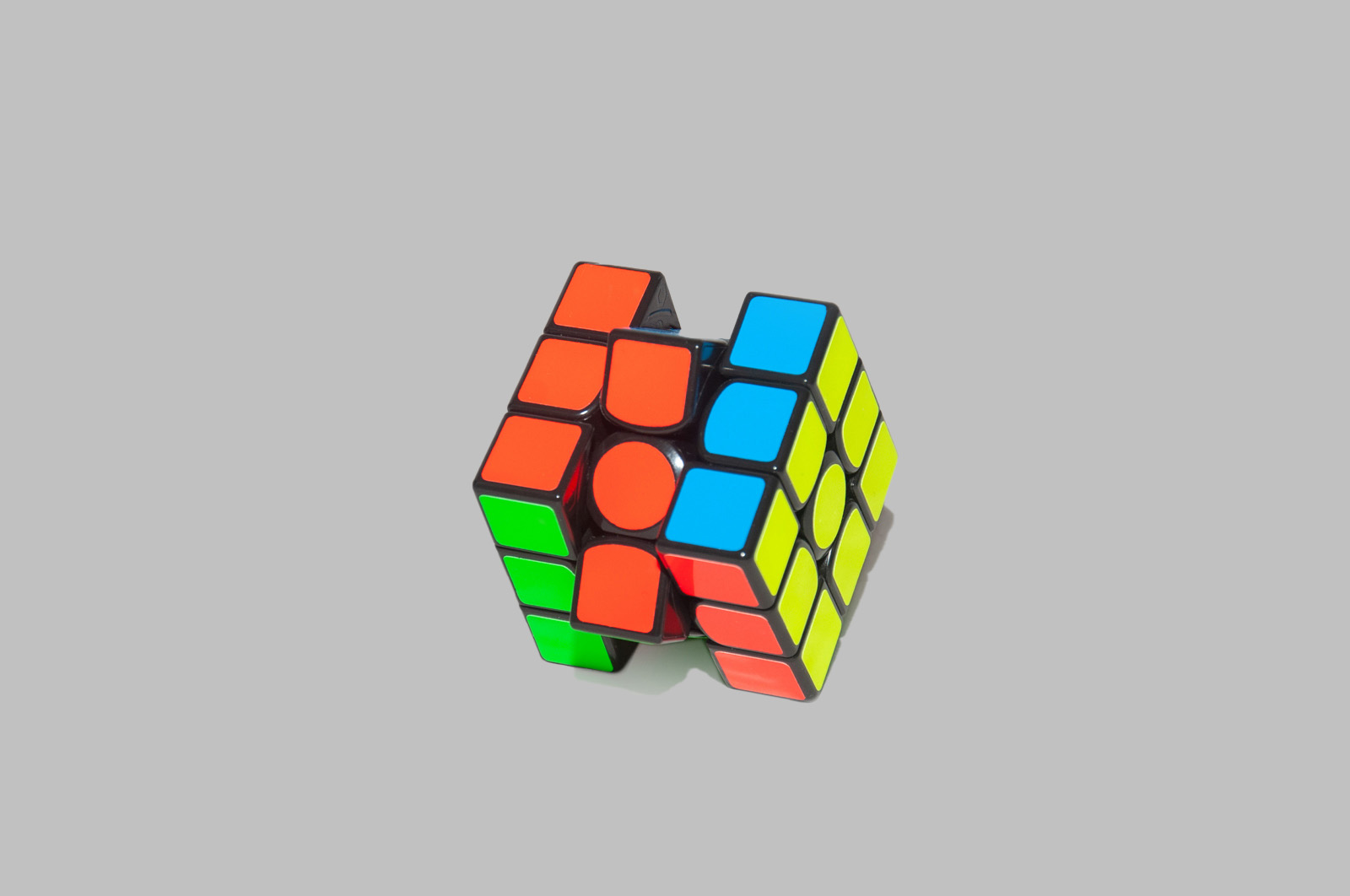# Magic Cube# GAN

## GAN

In mathematics, a magic cube is the 3-dimensional equivalent of a magic square, that is, a number of integers arranged in a n × n × n pattern such that the sums of the numbers on each row, on each column, on each pillar and on each of the four main space diagonals are equal to the same number, the so-called magic constant of the cube, denoted M3(n). It can be shown that if a magic cube consists of the numbers 1, 2, …, n3, then it has magic constant (sequence A027441 in the OEIS)

## Specification

Year of Production: 2017

Place of Production: China

Size: 8cm

Weight: 200g

Ace:
“Magic Cube was always curious in my life since I saw a friend of mine made a completion on it, when I was in university. So till 2017, I had spent two days to remember the formula, and finally, I successfully can make the completion of it.”